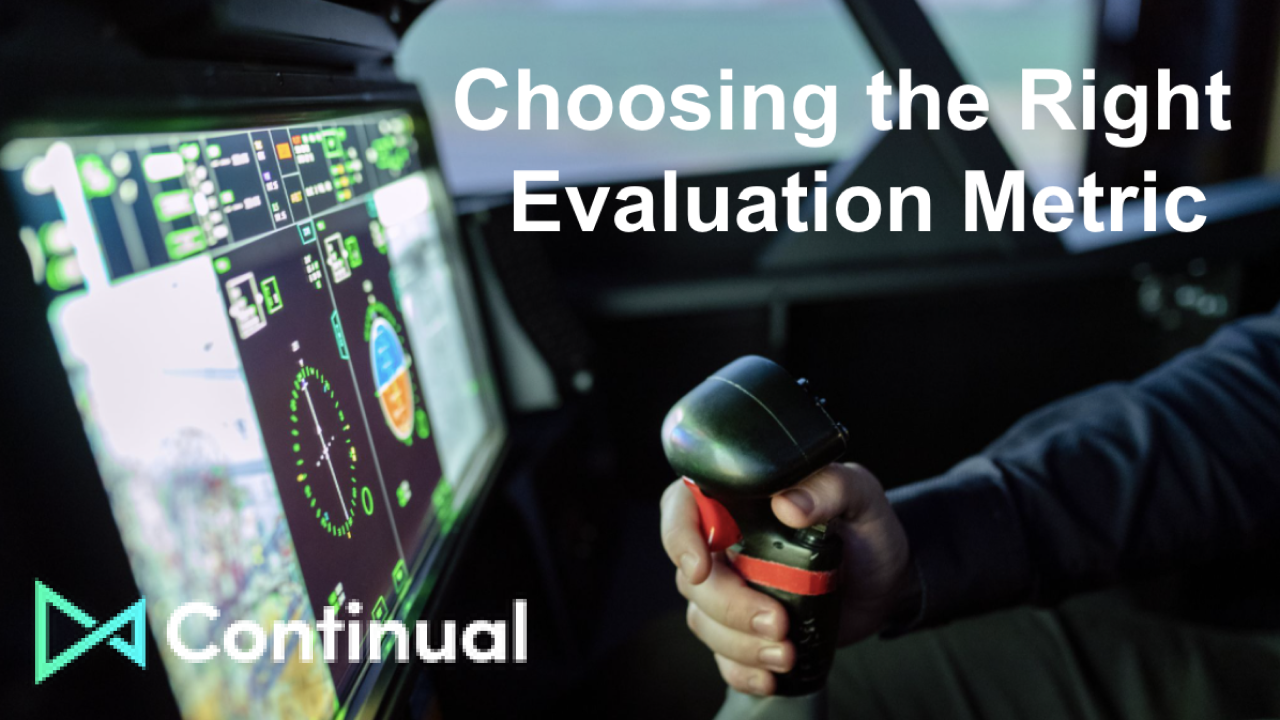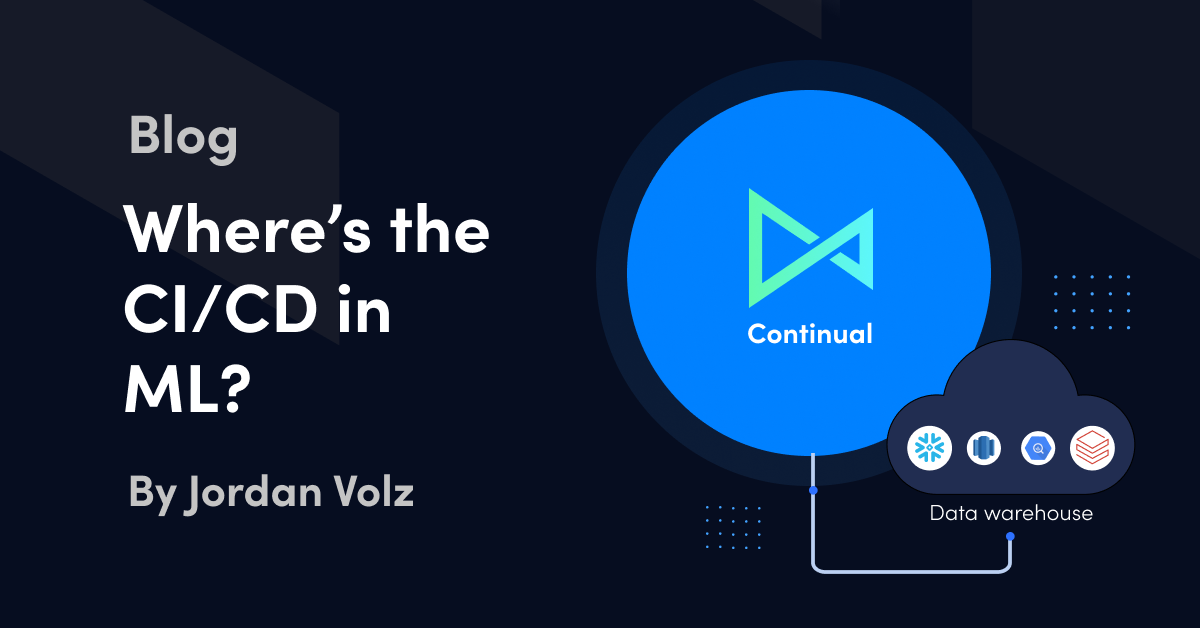# Choosing the Right Evaluation MetricMachine Learning

February 2, 2022

Is your model ready for production? It depends on how it’s measured. And measuring it with the right metric can unlock even better performance. Evaluating model performance is a vital step in building effective machine learning models. As you get started on Continual and start building models, understanding evaluation metrics helps to productionize the best performing model for your use case. While Continual’s modern gitops workflow and smooth dbt integration tend to attract attention from data teams, it’s also very important to learn how to use evaluation metrics correctly.

In this two part blog, we’ll cover Continual’s configurable evaluation metrics for optimizing and selecting the best performing model in Continual.

### What is an evaluation metric?

A model evaluation metric is a mechanism for assessing the performance of a machine learning model. A good metric should provide an informative summary of the error distribution. An ideal metric would be reliable, computationally and conceptually simple, scale-independent, outlier protected, and sensitive to changes. No single metric outperforms all others across this criteria. Therefore, it’s recommended to consider multiple metrics when selecting a model. By understanding the strengths and limitations of each metric, we are more likely to use them effectively.

Different problems require different classes of metrics. For example, for classification problems, accuracy can be a useful metric because we want to know how often the model predicts the correct class. But accuracy is not useful when making a value prediction for, say, a dollar amount or inventory supply because the target values are continuous and not discrete. Instead, for regression problems, we want to measure how close our predictions are to the actual values.

In part 1, we’ll cover 4 evaluation metrics used for regression problems. In part 2, we’ll cover the 4 main metrics for classification problems.

## Evaluation Metrics for Regression

### Mean Absolute Error (MAE)

The Mean Absolute Error (MAE) is simply the average distance between the model’s predicted values and the actual observed values. It disregards the direction of the error (whether it’s positive or negative) and computes the average distance. The lower the score the better. Its simplicity makes it easy to compute and to understand, even for business stakeholders which is a huge advantage. MAE is very popular for time series use cases. Nice and simple, right? What’s not to like?

Well, in its grand simplicity MAE treats every error the same no matter how small or how large. It’s hard to knock fairness but for use cases where extreme values are intolerable or even dangerous, another metric like RSME might be better suited. For example, a nuclear power plant device controller setting the temperature too high would be literally disastrous. But forecasting a music concert attendance too high wouldn’t be the end of the world.

When interpreting MAE it’s important to remember it’s scale-dependent; the size of the error is relative to the training data scale. This means interpreting the magnitude of the error depends on the context of the problem. For example, if you are predicting house prices then the MAE value would be in hundreds, tens of thousands, or millions of dollars. But if you’re predicting the volume of houses sold in a city then your MAE error will be in the hundreds or maybe thousands. It’s recommended for assessing model performance on a single problem series, but not adequate for comparing different series of different units.

For training models, the units we’re using aren’t terribly important because we only need to know whether we’re decreasing or increasing the error at each iteration. We care more about the relative size of the error. But when evaluating trained models, as you would on Continual, we do care about what units we’re using because we want to know whether the trained model is adequately solving our real-world problem.

MAE is a simple metric to evaluate the performance of your model but it’s important to be mindful of its scale-dependence and handling of outliers.

### Symmetric Mean Absolute Percentage Error (sMAPE)

To help us understand sMAPE, lets first talk about its predecessor: Mean Absolute Percentage Error (MAPE).

Just like MAE, MAPE calculates the absolute distance between the predicted values (F) and the actual values (A) but then divides the distance by the actual, outputting a percentage. Expressing the error as a percentage makes it easy to compare model performance across different scales. This is helpful for situations like forecasting inventory for various product SKUs. The business can rely on a consistent error percentage across different products of various price ranges, for example, a cheaper product like a laptop charger and a more expensive product like the laptop itself.

A problem with MAPE occurs when actual values are at, or close to, zero. In such a case, MAPE will output infinite or undefined values. Because zeros are common in many use cases, such as intermittent sales in a sales forecast, this is a severe limitation.

Symmetric MAPE was created to fix this problem by dividing the numerator by half the sum of the absolute values of the actual value (A) and the predicted value (F). Effectively, when either the actual or predicted value is at or close to zero, the sMAPE score will automatically hit its upper bound at 200%, instead of breaking.

The lower the score the better the prediction. But when interpreting the score, keep in mind it’s not perfectly symmetric. It penalizes positive errors (actual > predicted) more than negative errors (actual < predicted). For example, if our actual value = 100 and predicted value = 120 then sMAPE score is 18.2%. But if actual value = 100 and predicted value = 80, then sMAPE comes out to 22.2%.

Okay, so, we’ve covered sMAPE and MAE so far. Both are useful in cases where you aren’t worried about large errors. But when large errors are less tolerable, RMSE is your metric!

### Root Mean Squared Error (RMSE)

Suppose there's a model that predicts when the best time to deploy coolant in a nuclear power plant is. If the model forecasts too late, it could cause a literal disaster! In such a case, it's probably good to penalize large errors.

Whereas sMAPE and MAE penalize errors proportionally, Root Mean Squared Error (RMSE)  does it quadratically by squaring the error.

But that doesn’t mean the units of measure are squared. RMSE takes the square root of the error, effectively converting it back to the original scale. For example, if you’re working on a digital marketing use case and your target variable is using “impressions” as a unit, then the RMSE error will also have the “impressions” unit, not “squared impressions”. Pretty nifty, eh?

The last thing to know about RMSE is it’s dataset-dependent, so it shouldn’t be used to compare across datasets. It’s better used to measure the effectiveness of the model fit per dataset and reference MAE or sMAPE for comparison purposes.

R^2 Coefficient of Determination

R^2, also known as the coefficient of determination, is a ratio between the variance explained by the model and the total variance.

The numerator is calculated by the sum of the distance between the actual values and the best fitted line (model). The denominator is similar, but instead of finding the sum of the distance between the actual values and the best fitted line, we’re finding the distance to the mean.

The best possible score is 1.0 which means the model explains 100% of the variance and the fitted values would always equal the actual values. In other words, the independent variables explain all of the variance in the data. Conversely, if the model is zero it means it doesn’t explain any of the variance. R^2 can be negative because the model can be arbitrarily worse and fits the data worse than the mean.

When interpreting the R^2 error, be mindful of its propensity to be fooled. Just like any metric it has its vulnerability points and can give a high score for a bad model or a low score for a good model. It can be influenced by simply changing the range of our independent variables. And a low score might just be because it’s evaluating a non-linear model (R^2 is only valid for linear models). There's a lot of ways the metric can be wrong and consequently it's recommended to use other statistical measures to get a fuller view of your model.

Oh and don't forget R^2 is dataset-dependent (after all it is a measure of variance). It isn’t ideal to use to compare across series, just like RMSE isn't.

# Evaluation Metrics in Continual

Now that we’ve gone through the evaluation metrics for regression problems, let’s take a look at how they’re used in Continual.

Continual calculates multiple evaluation metrics and uses a default metric to compare performance by and select the best performing model.

In Model Overview, the evaluation metrics are displayed for the currently promoted model.

Model Version shows the metrics for each model trained and how the winning model compares to the previous version.

Users can set the default metric Continual will use to evaluate and select models in either the UI or CLI.

### Setting a default metric in the UI

When creating or editing a model, click the Performance Metric dropdown in the “Review Policies” step and choose the metric you want to use. When Continual trains, tests, and evaluates different models for your use case, it'll choose the model that performs best against the metric you set in the "Review Policies" step.

### Setting a default metric in the CLI

To define a default metric from the CLI, edit your model YAML file with the metric of your choosing. Here I’m using root mean squared error (RMSE) as my default metric, but alternatively I could’ve listed ‘mae’ or ‘r2’.

After updating the YAML, push the new model to Continual:

``continual push example.yml``

For any guidance on using the CLI, checkout our documentation.

# Part 2: Evaluation Metrics for Classification Problems

In our next post, we’ll discuss the evaluation metrics used for classification problems and show how users can build better performing classification models by using the right metric for their problem.

## Are you new to Continual?

Give it a whirl by signing up for a free trial.

## Sign up for more articles like this

Thank you! Your submission has been received!
Oops! Something went wrong while submitting the form.Machine Learning
###### Choosing the Right Evaluation Metric

Model performance depends on the metric being used. Understanding the strengths and limitation of each metric and interpreting the value correctly is key to building high performing models. In part 1, we cover four evaluation metrics commonly used for regression problems and demonstrate how to use them when building models on Continual.Feb 2, 2022Machine Learning
###### Where's the CI/CD in ML?

While many have called for stronger adherence to software development best practices in machine learning (ML) and artificial intelligence (AI) as well, today’s ML practitioners still lack simple tools and workflows to operate the ML deployment lifecycle on a level on par with software engineers. This article takes a trip down memory lane to explore the benefits of the CI/CD toolset and the detriment of their unfortunate absence in today’s ML development lifecycle.Dec 13, 2021